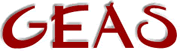[ < ] [ > ] [ << ] [ Up ] [ >> ] [Top] [Contents] [Index] [ ? ]

#### 1.2.11.1 The for statement

If you want a simple counter you should use the for statement. The syntax is as follows:

 ```for (initialize_statement; test_expression; end_of_loop_statement) body_statement; ```

When first entered, the `for` statement executes the initialize_statement part. This part usually is used to initialize counters or values used during the actual loop. Then the actual loop starts. Every loop starts by executing the test_expression and examining the result. This is a truth conditional, so any answer not equal to 0 will cause the loop to be run. If the answer is true the body_statement is executed, immediately followed by the end_of_loop_statement. In the body_statement you usually do what you want to have done during the loop, in the end_of_loop_statement you usually increment or decrement counters as needed.

Throughout the previous section I used the word usually a lot. This is because you don't have to do it that way, there's no rule forcing you to make use of the statements in the way I said. However, for now let's stick to the regular way of using the for-statement. Later on I'll describe more refined techniques.

Assume you want to compute the sum of all integers from 7 to 123 and don't know the formula ((x2^2 + x1^2) / 2). The easiest (if not most efficient) way of doing that is a loop.

 ```result = 0; for (count = 7; count < 124; count++) result += count; ```

What happens is that first of all result is set to 0, then the actual `for`-statement is entered. It begins by setting the variable count to 7. Then the loop is entered, beginning by testing if count (= 7) is less than 124, it is so the value is added to count. Then count is incremented one step and the loop entered again. This goes on until the count value reaches 124. Since that isn't less than 124 the loop is ended.

NB! The value of count after the `for`-statement will be 124 and not 123 that some people tend to believe. The test_expression must evaluate to `false` in order for the loop to end, and in this case the value for count then must be 124.

 [ < ] [ > ] [ << ] [ Up ] [ >> ] [Top] [Contents] [Index] [ ? ]

This document was generated by Ronny Wikh on July, 8 2003 using texi2html